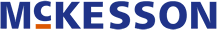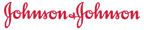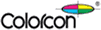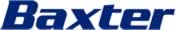➤ Get the DrugPatentWatch Daily Briefing

Get Daily Updates on Generic Entry, Litigation, Biosimilars, and more …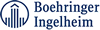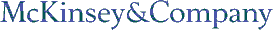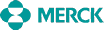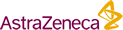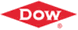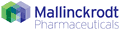Last Updated: October 22, 2021

DrugPatentWatch Database Preview

# Claims for Patent: 6,211,233

« Back to Dashboard

##### Summary for Patent: 6,211,233
 Title: Prostaglandin pharmaceutical compositions Abstract: Compounds of the general formula A--X.sub.1 --NO.sub.2, or their pharmaceutical compositions, wherein A contains a prostaglandin residue, X.sub.1 is a bivalent connecting bridge. Inventor(s): Del Soldato; Piero (Milan, IT) Assignee: Nicox S.A. (Paris, FR) Application Number: 09/423,286 Patent Claims: 1. Compounds of the formula wherein A is wherein t and u are integers and are equal to 0 or 1; X.dbd.O, NH, NR.sub.1c wherein R.sub.1c is a linear or branched alkyl having form 1 to 10 carbon atoms; R.sub.a and R.sub.b are equal to or different from each other and are H, or a C.sub.1 -C.sub.3 alkyl radical and R is a radical having the following formula: ##STR10## wherein m.sub.0 is an integer having a value of 0 or 1; and when t=1, u=0 and m.sub.0 =1: R.sub.1 =H; an alkyl having from 1 to 6 carbon atoms or a free valance; R.sub.2 =OH, O-- such as to form with R.sub.1 when R.sub.1 is a free valance, and with the carbon atom at position 15 a C.dbd.O group; R.sub.3 and R.sub.4 are equal to or different from one another and are equal to R.sub.1 or one of them is a bond O--, and the other is a free valance so that with the carbon atom C.sub.6 they form a C.dbd.O group; R.sub.5 and R.sub.6 are equal to or different from one another, and are equal to R.sub.1 when both R.sub.5 and R.sub.3 are each a free valence, R.sub.5 and R.sub.3 form a double bond between C.sub.5 and C.sub.6 ; R.sub.7, R.sub.8, R.sub.9, and R.sub.10 are equal to or different from each other, and have the same meaning as R.sub.1 ; when R.sub.7 or R.sub.9, and at the same time R.sub.8 or R.sub.10 are each a free valence, there is a double bond between C.sub.13 and C.sub.14 ; R.sub.11 =R.sub.1 ; R.sub.12 =R.sub.11 or OH; R.sub.13, R.sub.14, R.sub.15, and R.sub.16 are equal to or different from each other, and are equal to R.sub.1 ; when R.sub.13 or R.sub.15, and at the same time R.sub.14 or R.sub.16, are each a free valence, there is a double bond between C.sub.3 and C.sub.2 ; R.sub.17 and R.sub.18 are equal to or different from each other and are equal to R.sub.1 ; R.sub.19 and R.sub.20 are equal to or different from each other and are equal to R.sub.1 ; when R.sub.6 or R.sub.5 is a free valence, and R.sub.19 or R.sub.20 is a free valence, there is a double bond between C.sub.4 and C.sub.5 ; R.sub.21, R.sub.22, R.sub.23, and R.sub.24 are equal to or different from each other and are equal to R.sub.1 ; R.sub.25 and R.sub.26 are equal to or different from each other and are equal to R.sub.1, but both R.sub.25 and R.sub.26 cannot be a free valence, R.sub.27 is a linear or branched alkyl having one to six carbon atoms; B is equal to the group O-- (a keto group with the carbon atom at position 9 of the prostaglandin molecule) or is OH or --O--; when no aliphatic chain C.sub.7 -C.sub.2 is at position 8, there is in its place an alkylaromatic residue: ##STR11## which is bound to formula (III) (B=--O--) in the following way: ##STR12## wherein m.sub.1 is an integer from 1 to 6, R.sub.a and R.sub.b are equal to or different from each other, and are as defined above, when t=0, u=1, and m.sub.0 =1 the meanings of the various substituents are as defined above, when t=1, u=0, and m.sub.0 =0 the meanings of the various substituents are as defined above and C.sub.16 is bound, optionally by a bridging group --O--, to an aromatic radical or an alkyl-aryl radical, where the aryl can be substituted, said aryl radical can also contain heteroatoms, such as O or N; the alkyl of the alkyl-aryl radical is an aliphatic chain from 1 to 3 carbon atoms; X.sub.1 of formula A--X.sub.1 --NO.sub.2 is a bivalent connecting bridge, chosen from the following: where Y is a linear or whenever possible branched C.sub.1 -C.sub.20 alkylene oxygen terminated, or is a C.sub.5 -C.sub.7 cycloalkylene oxygen terminated optionally substituted; ##STR13## where n.sub.3 is an integer from 0 to 3; ##STR14## where nf' is an integer from 1 to 6; and ##STR15## where R.sub.1f =H or CH.sub.3, and nf is an integer from 1 to 6. 2. Compounds according to claim 1 wherein the prostaglandin residues R are the following: when in formula (II) t=1 u=0 and in formula (III) m.sub.0 =1: B is O.dbd. (keto group with C.sub.9); R.sub.7, R.sub.8, R.sub.9 and R.sub.10 are such as to given a double bond between C.sub.13 and C.sub.14 ; R.sub.2 is OH; R.sub.27 is CH.sub.3 ; the substituents of the carbon atoms of the C.sub.2 -C.sub.7 and C.sub.16 -C.sub.19 aliphatic chains are H; R thus defined is known as the residue of Prostaglandin E.sub.1 ; or, by putting in the formula of Prostaglandin E.sub.1, R.sub.1 =CH.sub.3 and R.sub.3, R.sub.4, R.sub.5, R.sub.6 such as to give a double bond between C.sub.5 and C.sub.6 ; R thus defined is known as the residue of Arbaprostil; or, by putting in the formula of Arbaprostil R.sub.7 =R.sub.8 =R.sub.9 =R.sub.10 =H; R.sub.1 and R.sub.2 are such as to form the group C.dbd.O with C.sub.15 ; B is OH; R.sub.27 =C.sub.3 H.sub.7 ; R thus defined is known as the residue of Unoprostone; or, by putting in the formula of Arbaprostil R.sub.11 =R.sub.12 =CH.sub.3, R.sub.1 =H, R thus defined s known as the residue of Trimoprostil; or, when in the formula of Arbaprostil B is OH; R.sub.1 =H; R thus defined is known as the residue of Prostaglandin F.sub.2.alpha. ; or, when in the formula of Prostaglandin F.sub.2.alpha. B is O.dbd. (keto group with C.sub.9); R thus defined is known as the residue of Prostaglandin R.sub.2 ; or, when in the formula of Arbaprostil B is OH; R thus defined is known as the residue of Carboprost; or, by putting in the formula of Arbaprostil R.sub.1 =H; R.sub.17 =H; R.sub.19 =CH.sub.3 ; R.sub.3 =R.sub.4 =R.sub.5 =R.sub.6 =H; R.sub.27 =C.sub.2 H.sub.5 ; R.sub.13 =R.sub.16 =H and R.sub.14 =R.sub.15 being free valences such as to form a double bond between C.sub.2 and C.sub.3 ; R thus defined is known as the residue of Limaprost; or, by putting in the formula of Trimoprostil R.sub.3 =R.sub.4 =R.sub.5 =R.sub.6 =H, and positioning the double bond between C.sub.2 and C.sub.3 instead that between C.sub.5 and C.sub.6 ; R thus defined is known as the residue of Gemeprost; or, by putting in the formula of Arbaprostil R.sub.1 =R.sub.2 =H; R.sub.12 =OH; R.sub.11 =CH.sub.3, R.sub.3 =R.sub.5 =R.sub.4 =R.sub.6 =H; R thus defined is known as the residue of Misoprost; or, by putting in the formula of Arbaprostil R.sub.1 =H; R.sub.18 =CH.sub.3 ; R.sub.27 =C.sub.2 H.sub.5 ; R.sub.3 and R.sub.4 are such that one of them is a free valence and the other is a single bond with an oxygen atom so that together with the carbon atom C.sub.6 form a keto group C.dbd.O; R5=R6=H, R thus defined is known as the residue of Ornoprostil; or, as in Arbaprostil, without the C.sub.7 -C.sub.2 aliphatic chain and the carbon atoms C.sub.9 -C.sub.8 linked to the group of formula (IV) as shown in (V); R.sub.1 =H; R.sub.11 =CH.sub.3 ; R.sub.21, R.sub.22, R.sub.23, R.sub.24 being each a free valence to form a triple bond between C.sub.18 and C.sub.19 ; R thus defined is known as the residue of Beraprost; when t=0; u=1 and m.sub.0 =1: R.sub.a =R.sub.b =H and R is the residue of Misoprostol, R thus defined is known as the residue of Rioprostil, when t=1, u=0 and m.sub.0 =0: when R is the residue of Arbaprostil except that R.sub.1 =H; R.sub.19 or R.sub.20 is a free valence or H, so that between C.sub.4 and C.sub.5 there is a double bond; C.sub.16 is linked to a group --O--A.sub.r wherein A.sub.r =phenyl; R thus defined is known as the residue of Enprostil; when R is the residue of Arbaprostil except that B is OH; R.sub.1 =H; C.sub.16 is linked to a group --CH.sub.2 --A.sub.r where A.sub.r is phenyl; it is defined a radical known as the residue of Latanaprost; when in the formula of Enprostil R.sub.20 =R.sub.19 =H; it is defined a radical known as the residue of Sulprostone. 3. A compound according to claim 1 where R is the residue of Prostaglandin E.sub.1. 4. A method for treating cerebrovascular and cardiovascular disorders, glaucoma, and peptic ulcer disorders, and a method for inducing abortion, comprising administering to a patient an effective amount of a compound of claim 1. 5. Pharmaceutical compositions containing as the active ingredient compounds of claim 1 and a pharmaceutically acceptable carrier. 6. A method for treating impotence comprising administering to a patient an effective amount of a compound of the formula: wherein A is wherein t and u are integers and are equal to 0 or 1; X.dbd.O, NH, NR.sub.1c wherein R.sub.1c is a linear or branched alkyl having form 1 to 10 carbon atoms; R.sub.a and R.sub.b are equal to or different from each other and are H, or a C.sub.1 -C.sub.3 alkyl radical and R is a radical having the following formula: ##STR16## wherein m.sub.0 is an integer having a value of 0 or 1; and when t=1, u=0 and m.sub.0 =1: R.sub.1 =H; an alkyl having from 1 to 6 carbon atoms or a free valance; R.sub.2 =OH, O-- such as to form with R.sub.1 when R.sub.1 is a free valance, and with the carbon atom at position 15 a C.dbd.O group; R.sub.3 and R.sub.4 are equal to or different from one another and are equal to R.sub.1 or one of them is a bond O--, and the other is a free valance so that with the carbon atom C.sub.6 they form a C.dbd.O group; R.sub.5 and R.sub.6 are equal to or different from one another, and are equal to R.sub.1 when both R.sub.5 and R.sub.3 are each a free valence, R.sub.5 and R.sub.3 form a double bond between C.sub.5 and C.sub.6 ; R.sub.7, R.sub.8, R.sub.9, and R.sub.10 are equal to or different from each other, and have the same meaning as R.sub.1 ; when R.sub.7 or R.sub.9, and at the same time R.sub.8 or R.sub.10 are each a free valence, there is a double bond between C.sub.13 and C.sub.14 ; R.sub.11 =R.sub.1 ; R.sub.12 =R.sub.11 or OH; R.sub.13, R.sub.14, R.sub.15, and R.sub.16 are equal to or different from each other, and are equal to R.sub.1 ; when R.sub.13 or R.sub.15, and at the same time R.sub.14 or R.sub.16, are each a free valence, there is a double bond between C.sub.3 and C.sub.2 ; R.sub.17 and R.sub.18 are equal to or different from each other and are equal to R.sub.1 ; R.sub.19 and R.sub.20 are equal to or different from each other and are equal to R.sub.1 ; when R.sub.6 or R.sub.5 is a free valence, and R.sub.19 or R.sub.20 is a free valence, there is a double bond between C.sub.4 and C.sub.5 ; R.sub.21, R.sub.22, R.sub.23, and R.sub.24 are equal to or different from each other and are equal to R.sub.1 ; R.sub.25 and R.sub.26 are equal to or different from each other and are equal to R.sub.1, but both R.sub.25 and R.sub.26 cannot be a free valence, R.sub.27 is a linear or branched alkyl having one to six carbon atoms; B is equal to the group O.dbd. (a keto group with the carbon atom at position 9 of the prostaglandin molecule) or is OH or --O--; when no aliphatic chain C.sub.7 -C.sub.2 is at position 8, there is in its place an alkylaromatic residue: ##STR17## which is bound to formula III (B=--O--) in the following way: ##STR18## wherein m.sub.1 is an integer from 1 to 6, R.sub.a and R.sub.b, are equal to or different from each other, and are as defined above, when t=0, u=1, and m.sub.0 =1 the meanings of the various substituents are as defined above, when t=1, u=0, and m.sub.0 =0 the meanings of the various substituents are as defined above and C.sub.16 is bound, optionally by a bridging group --O--, to an aromatic radical or an alkyl-aryl radical, where the aryl can be substituted, said aryl radical can also contain heteroatoms, such as O or N; the alkyl of the alkyl-aryl radical is an aliphatic chain from 1 to 3 carbon atoms; X.sub.1 of formula A--X.sub.1 --NO.sub.2 is a bivalent connecting bridge, chosen from the following: where Y is a linear or whenever possible branched C.sub.1 -C.sub.20 alkylene oxygen terminated, or is a C.sub.5 -C.sub.7 cycloalkylene oxygen terminated optionally substituted; ##STR19## where n.sub.3 is an integer from 0 to 3; ##STR20## ##STR21## where nf' is an integer from 1 to 6; and ##STR22## where R.sub.1f =H or CH.sub.3, and nf is an integer from 1 to 6.

### Make Better Decisions: Try a trial or see plans & pricing